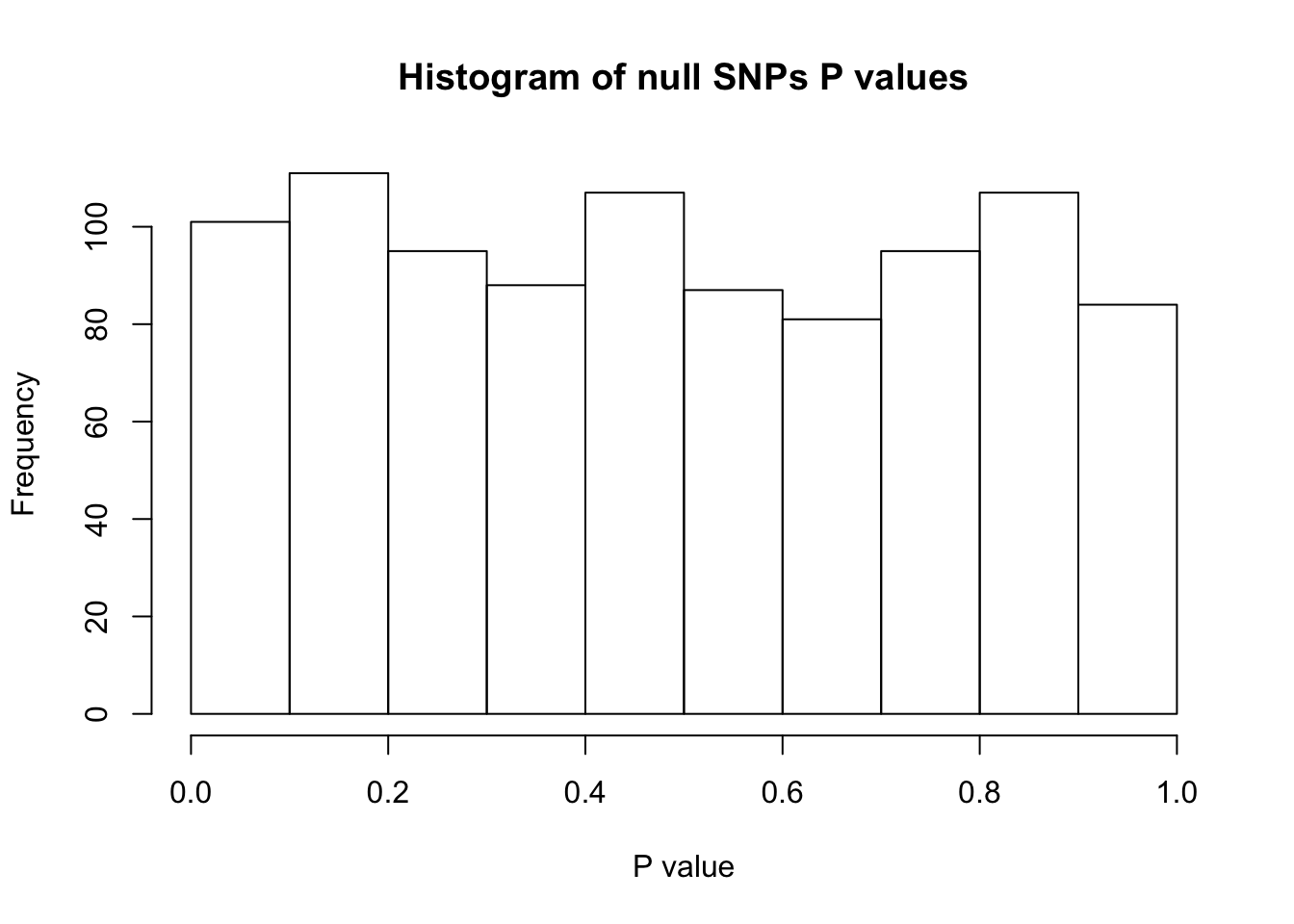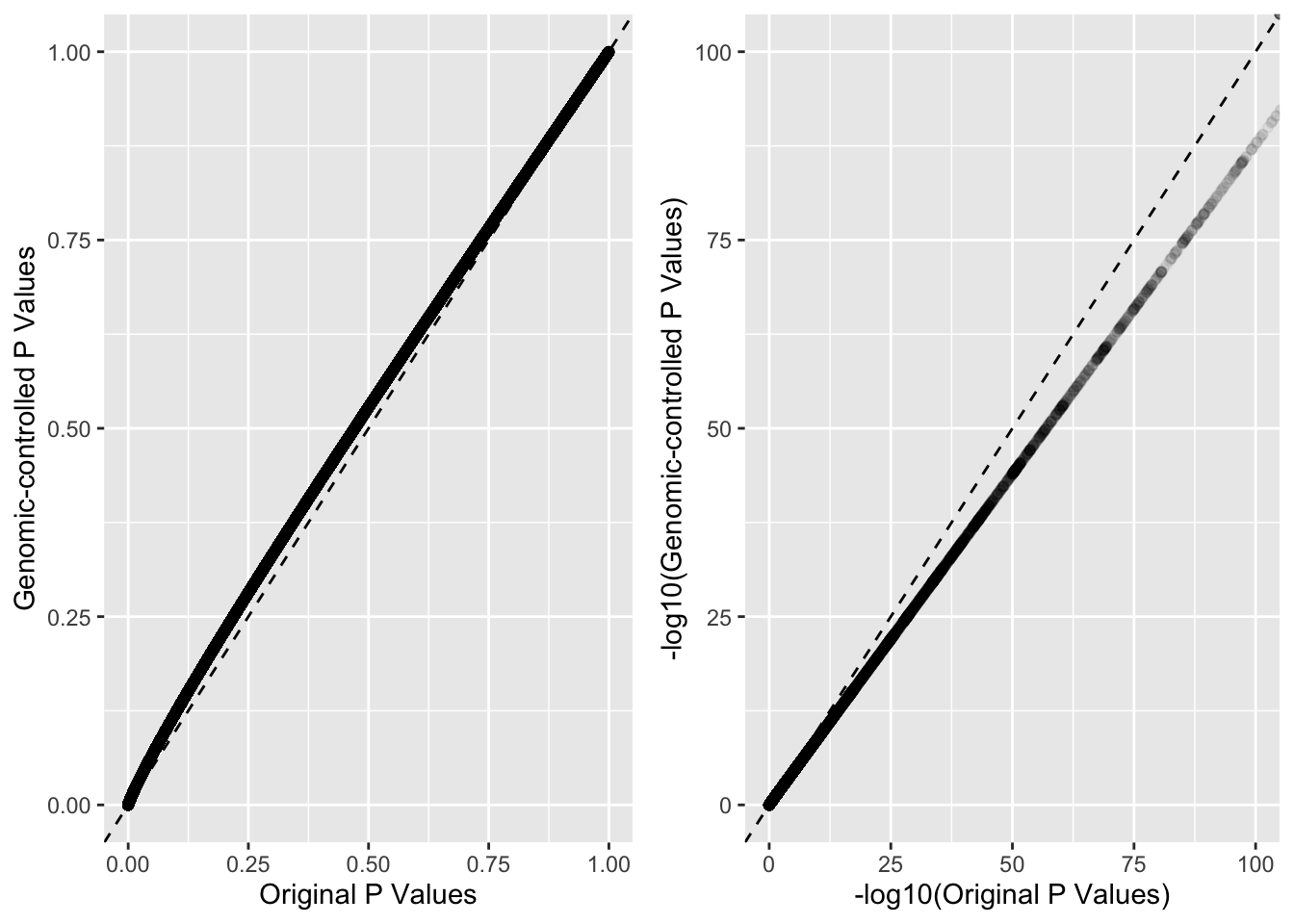Population stratification and relatedness may lead to the inflation of test statistic in GWAS and should be controlled for. Genomic control evaluates whether or not the population structure exists and in the presence of population stucture, assumes that the chi-squared statistic $$\chi^2$$ is inflated by a constant inflation factor $$\lambda$$, which is defined as the empirical median of L unrelated statistics divided by the expected median under the null distibution .

#### Example

I assume that the P values in the stats-t1d.csv file have not been genomic-controlled. To perform genomic-control I need some ‘null SNPs’ that are not associated with T1D. Luckily, the ImmunoChip contained variants associated with reading ability, which I use as my ‘null SNPs’.

• The null SNP positions are hg18 and the MyData (stats-t1d.csv) SNP positions are hg19. Rather than lifting over, for now I just match by rsID (but should use liftover at some point as I can only match 956 out of 1200 by name).
stats <- readRDS("MyData.RDS")

nullSNPs$p_stats <- stats[match(nullSNPs$rsid, stats$snp),"p"] nullSNPs <- na.omit(nullSNPs) hist(nullSNPs$p_stats, xlab = "P value", main = "Histogram of null SNPs P values")# chi-square statistic for T1D
qchi <- qchisq(nullSNPs$p_stats, df=1, lower.tail=FALSE) A <- median(qchi) A ##  0.5193442 1. Find the expected median under the null distribution (i.e. of the null SNPs). # expected median B <- qchisq(0.5, df=1, lower.tail=FALSE) B ##  0.4549364 1. Find the inflation factor, $$\lambda$$. # inflation factor lambda lambda <- A/B lambda  ##  1.141575 (Note that $$\lambda$$ is usually approx. 1.05 so this value seems a little extreme) 1. Divide chi squared statistics of T1D SNPs by lambda and find genomic-controlled P values. chi_p <- qchisq(stats$p, df=1, lower.tail=FALSE)
stats\$GC_P <- pchisq(chi_p/lambda, df=1, lower.tail=FALSE)